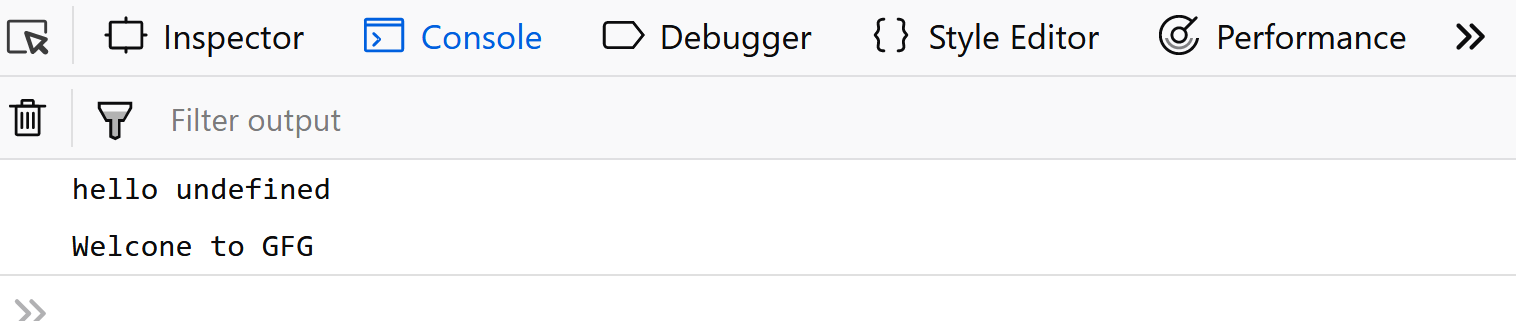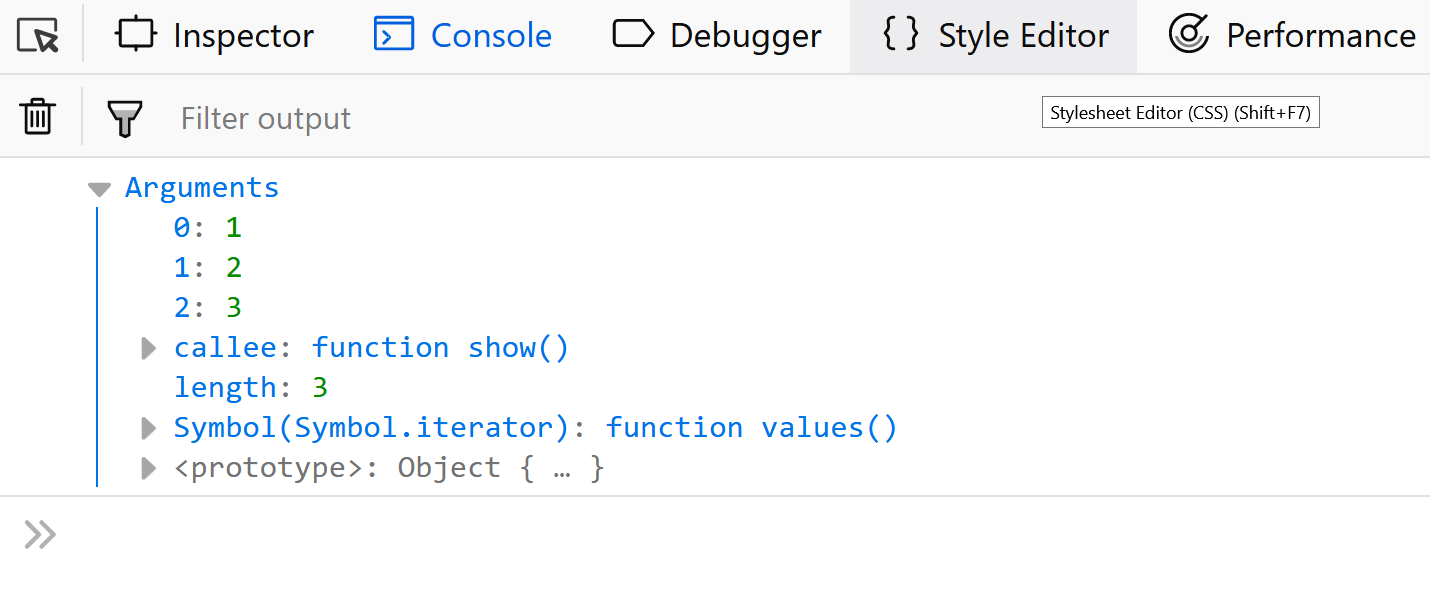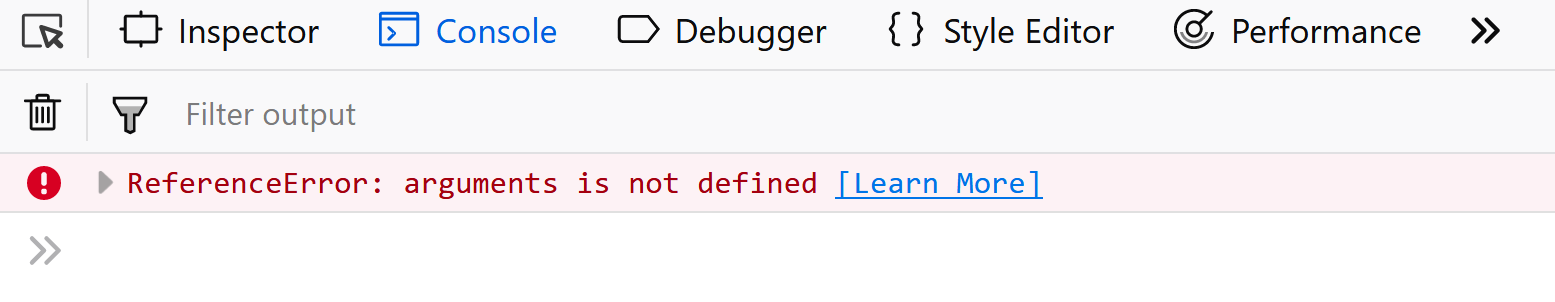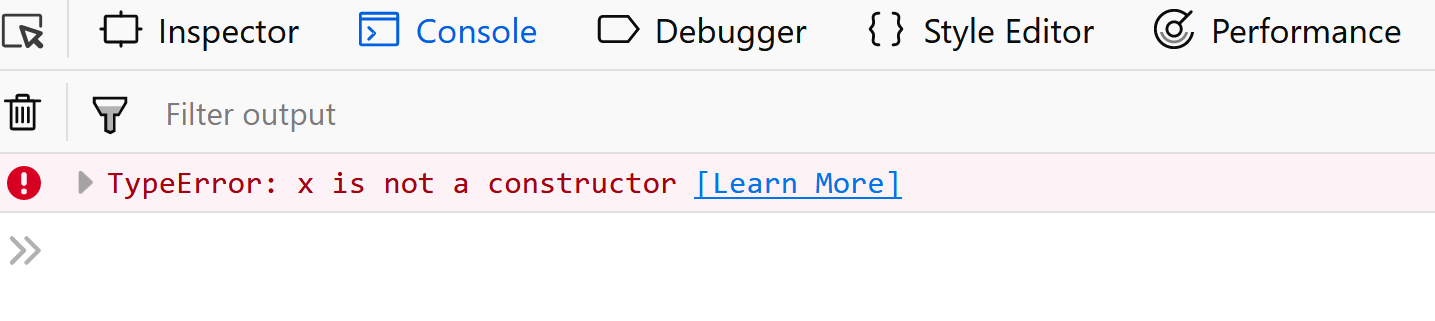# Difference between regular functions and arrow functions

• Difficulty Level : Basic
• Last Updated : 24 May, 2019

This article discusses the major differences between the regular functions and the arrow functions.

Arrow functions – a new feature introduced in ES6 – enable writing concise functions in JavaScript. While both regular and arrow functions work in a similar manner, yet there are certain interesting differences between them, as discussed below.

Syntax

Syntax of regular functions:-

 `let x = ``function` `function_name(parameters){ ` `   ``// body of the function ` `}; `

Example of regular functions:-

 `let square = ``function``(x){ ` `  ``return` `(x*x); ` `}; ` `console.log(square(9)); `

Output:The syntax of arrow functions:-

 `let x = (parameters) => { ` `    ``// body of the function ` `}; `

Example of arrow functions:-

 `var` `square = (x) => { ` `    ``return` `(x*x); ` `}; ` `console.log(square(9)); `

Output:Use of this keyword

Unlike regular functions, arrow functions do not have their own `this`.
For example:-

 `let user = { ` `    ``name: ``"GFG"``, ` `    ``gfg1:() => { ` `        ``console.log(``"hello "` `+ ``this``.name); ``// no 'this' binding here ` `    ``}, ` `    ``gfg2(){        ` `        ``console.log(``"Welcome to "` `+ ``this``.name); ``// 'this' binding works here ` `    ``}   ` ` ``}; ` `user.gfg1(); ` `user.gfg2(); `

Output:Availability of `arguments` objects

Arguments objects are not available in arrow functions, but are available in regular functions.

Example using regular ():-

 `let user = {       ` `    ``show(){ ` `        ``console.log(arguments); ` `    ``} ` `}; ` `user.show(1, 2, 3); `

Output:Example using arrow ():-

 `let user = {      ` `        ``show_ar : () => { ` `        ``console.log(...arguments); ` `    ``} ` `}; ` `user.show_ar(1, 2, 3); `

Output:Using `new` keyword

Regular functions created using function declarations or expressions are ‘constructible’ and ‘callable’. Since regular functions are constructible, they can be called using the ‘new’ keyword. However, the arrow functions are only ‘callable’ and not constructible. Thus, we will get a run-time error on trying to construct a non-constructible arrow functions using the new keyword.

Example using regular function:-

 `let x = ``function``(){ ` `    ``console.log(arguments); ` `}; ` `new` `x =(1,2,3); `

Output:Example using arrow function:-

 `let x = ()=> { ` `    ``console.log(arguments); ` `}; ` `new` `x(1,2,3); `

Output: